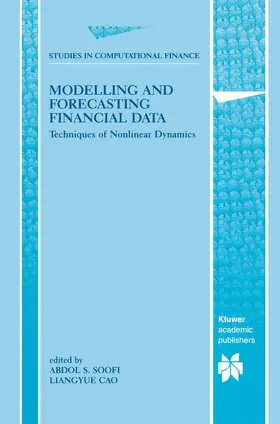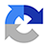# Soofi / Liangyue CaoModelling and Forecasting Financial Data

Techniques of Nonlinear Dynamics

2002, Band: 2, 488 Seiten, Gebunden, Book, Format (B × H): 162 mm x 246 mm, Gewicht: 1990 g Reihe: Studies in Computational Finance
ISBN: 978-0-7923-7680-4
Verlag: Springer-Verlag GmbH

## Soofi / Liangyue CaoModelling and Forecasting Financial Data

Modelling and Forecasting Financial Data brings together a coherent and accessible set of chapters on recent research results on this topic. To make such methods readily useful in practice, the contributors to this volume have agreed to make available to readers upon request all computer programs used to implement the methods discussed in their respective chapters.
Modelling and Forecasting Financial Data is a valuable resource for researchers and graduate students studying complex systems in finance, biology, and physics, as well as those applying such methods to nonlinear time series analysis and signal processing.

Research

## Weitere Infos & Material

List of Figures. List of Tables. Preface. Contributing Authors. Introduction; A.S. Soofi, Liangyue Cao. Part I: Embedding Theory: Time-Delay Phase Space Reconstruction and Detection of Nonlinear Dynamics. 1. Embedding Theory: Introduction and Applications to Time Series Analysis; F. Strozzi, J.M. Zaldivar. 2. Determining Minimum Embedding Dimension; Liangyue Cao. 3. Mutual Infomation and Relevant Variables for Predictions; B. Pompe. Part. II: Methods of Nonlinear Modelling and Forecasting. 4. State Space Local Linear Prediction; D. Kugiumtzis. 5. Local Polynomial Prediction and Volatility Estimation in Financial Time Series; Zhan-Qian Lu. 6. Kalman Filtering of Time Series Data; D.M. Walker. 7. Radial Basis Functions Networks; A. Braga, et al. 8. Nonlinear Prediction of Time Series Using Wavelet Network Method; Liangyue Cao. Part III: Modelling and Predicting Multivariate and Input-Output Time Series. 9. Nonlinear Modelling and Prediction of Multivariate Financial Time Series; Liangyue Cao. 10. Analysis of Economic Time Series Using NARMAX Polynomial Models; L.A. Aquirre, A. Aguirre. 11. Modeling dynamical systems by Error Correction Neural Networks; H.-G. Zimmermann, et al. Part IV: Problems in Modelling and Prediction. 12. Surrogate Data Test on Time Series; D. Kugiumtzis. 13. Validation of Selected Global Models; C. Letellier. 14. Testing Stationarity in Time Series; A. Witt, J. Kurths. 15. Analysis of Economic Delayed-Feedbak Dynamics; H.U. Voss, J. Kurths. 16. Global Modeling and Differential Embedding; J. Maquet, et al. 17. Estimation of Rules Underlying Fluctuating Data; S. Siegert, et al. 18. Nonlinear Noise Reduction; R. Hegger, et al. 19. Optimal Model Size; Jianming Ye. 20. Influence of Measured Time Series in the Reconstruction of Nonlinear Multivariable Dynamics; C. Letellier, L.A. Aguirre. Part. V: Applications in Economics and Finance. 21. Nonlinear Forecasting of Noisy Financial Data; A.S. Soofi, L. Cao. 22. Canonical Variate Analysis and its Applications to Financial Data; B. Pilgram, et al. Index.

## Ihre Fragen, Wünsche oder Anmerkungen

Ihre Nachricht*
Wie möchten Sie kontaktiert werden?
Anrede*
Titel
Vorname*
Nachname*